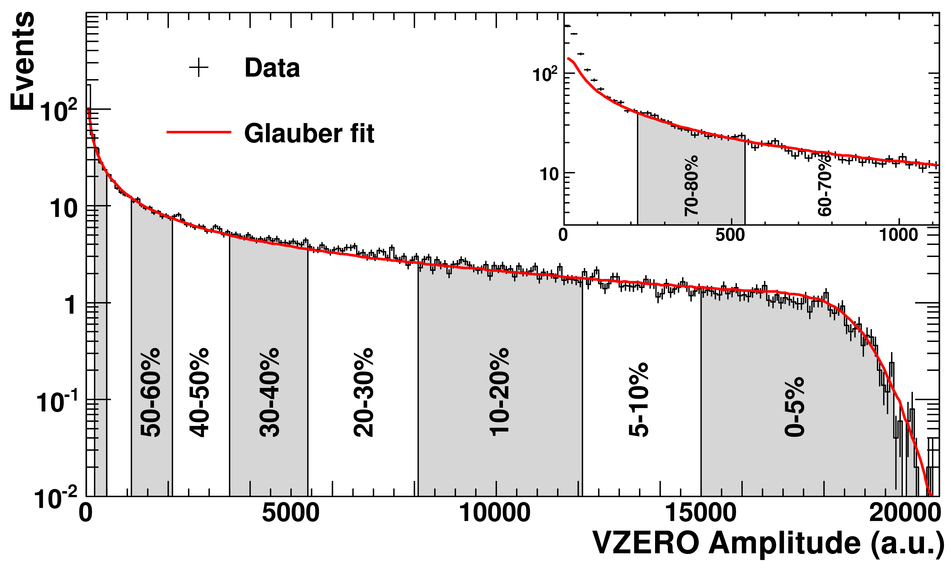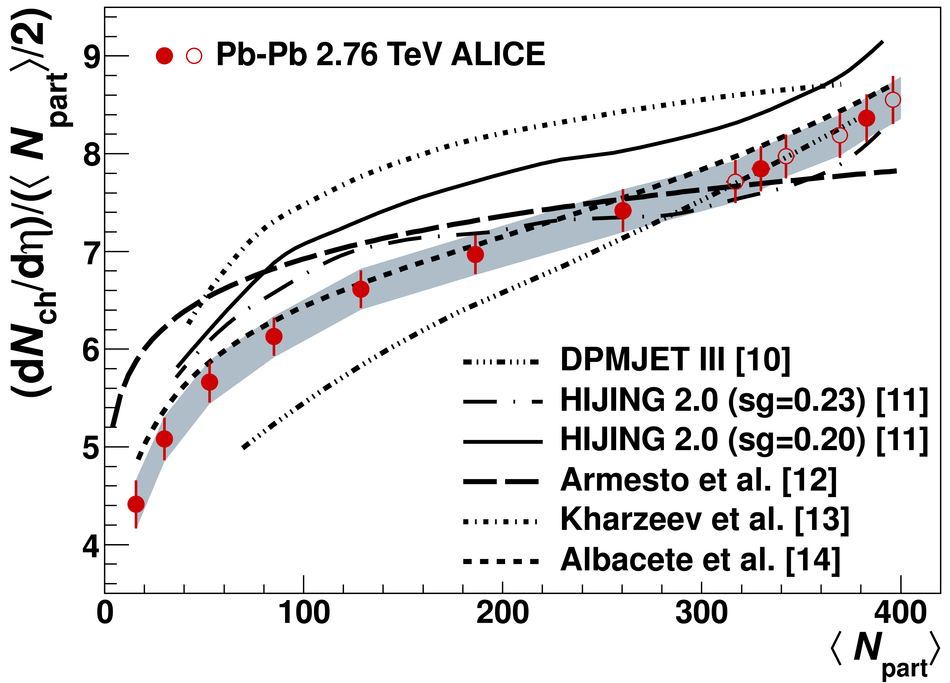# Centrality dependence of the charged-particle multiplicity density at mid-rapidity in Pb-Pb collisions at $\sqrt{s_{\rm NN}}$ = 2.76 TeV

The centrality dependence of the charged-particle multiplicity density at mid-rapidity in Pb-Pb collisions at $\sqrt{s_{\rm NN}}$ = 2.76 TeV is presented. The charged-particle density normalized per participating nucleon pair increases by about a factor 2 from peripheral (70-80%) to central (0-5%) collisions. The centrality dependence is found to be similar to that observed at lower collision energies. The data are compared with models based on different mechanisms for particle production in nuclear collisions.

Figures

## Figure 1

 Distribution of the summed amplitudes in the VZERO scintillator tiles (histogram); inset shows the low amplitude part of the distribution The curve shows the result of the Glauber model fit to the measurement The vertical lines separate the centrality classes used in the analysis, which in total correspond to the most central 80% of hadronic collisions.## Figure 2

 Dependence of (d$N_{\rm ch}$/d$\eta)$ / ($\langle N_{\rm part} \rangle$ / 2) on the number of participants for Pb-Pb collisions at $\snn = 2.76$ TeV and Au-Au collisions at $\snn=0.2$ TeV (RHIC average) The scale for the lower-energy data is shown on the right-hand side and differs from the scale for the higher-energy data on the left-hand side by a factor of 2.1 For the Pb-Pb data, uncorrelated uncertainties are indicated by the error bars, while correlated uncertainties are shown as the grey band Statistical errors are negligible The open circles show the values obtained for centrality classes obtained by dividing the 0-10% most central collisions into four, rather than two classes The values for non-single-diffractive and inelastic pp collisions are the results of interpolating between data at 2.36 and 7 TeV.## Figure 3

 Comparison of (d$N_{\rm ch}$/d$\eta)$ / ($\langle N_{\rm part} \rangle$ / 2) with model calculations for Pb-Pb at $\snn=2.76~$TeV Uncertainties in the data are shown as in Fig. 2 The HIJING 2.0 curve is shown for two values of the gluon shadowing ($s_{g}$) parameter.